# hittime

Compute Markov chain hitting times

## Syntax

``ht = hittime(mc,target)``
``ht = hittime(mc,target,'Graph',true)``
``[ht,h] = hittime(mc,target,'Graph',true)``
``[ht,h] = hittime(ax,mc,target,'Graph',true)``

## Description

example

``ht = hittime(mc,target)` returns the expected first hitting time `ht` for a specified subset of states `target`, beginning from each state in the Markov chain `mc`. If `target` forms a recurrent class, the elements of `ht` are expected absorption times.`

example

``ht = hittime(mc,target,'Graph',true)` plots a directed graph of `mc` with node colors representing the expected first hitting times. A color bar summarizes the color coding.`
````[ht,h] = hittime(mc,target,'Graph',true)` also returns the plot handle. Use `h` to modify properties of the plot after you create it.```
``` `[ht,h] = hittime(ax,mc,target,'Graph',true)` plots on the axes specified by `ax` instead of the current axes (`gca`).```

## Examples

collapse all

Consider this theoretical, right-stochastic transition matrix of a stochastic process.

`$P=\left[\begin{array}{cccc}1& 0& 0& 0\\ 1/2& 0& 1/2& 0\\ 0& 0& 0& 1\\ 0& 0& 1/2& 1/2\end{array}\right].$`

Create the Markov chain that is characterized by the transition matrix P.

```P = [1 0 0 0; 1/2 0 1/2 0; 0 0 0 1; 0 0 1/2 1/2]; mc = dtmc(P);```

Plot a directed graph of the Markov chain. Visually identify the communicating class to which each state belongs by using node colors.

```figure; graphplot(mc,'ColorNodes',true);```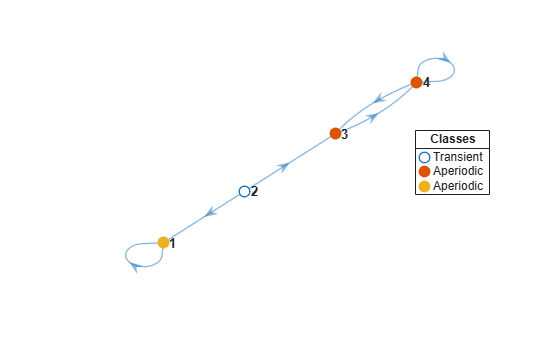Compute the expected first hitting time for state 3, beginning from each state in the Markov chain.

`ht = hittime(mc,3)`
```ht = 4×1 Inf Inf 0 2 ```

Because state 3 is unreachable from state 1, state 1 is a remote state with respect to state 3, with an expected first hitting time of `Inf`.

State 3 is reachable from state 2, but state 2 has a positive probability of transitioning to state 1, which is an absorbing state. Therefore, state 2 is a remote-reachable state with respect to state 3, with an expected first hitting time of `Inf`.

Because state 3 is the target, its expected first hitting time for itself is `0`.

The expected first hitting time for state 3 starting from state 4 is `2` time steps.

Consider this theoretical, right-stochastic transition matrix of a stochastic process.

`$P=\left[\begin{array}{cccc}0& 1/2& 0& 1/2\\ 2/3& 0& 1/3& 0\\ 0& 1/2& 0& 1/2\\ 1/3& 0& 2/3& 0\end{array}\right].$`

Create the Markov chain that is characterized by the transition matrix P.

```P = [0 1/2 0 1/2 2/3 0 1/3 0 0 1/2 0 1/2 1/3 0 2/3 0 ]; mc = dtmc(P);```

Plot a digraph of the Markov chain `mc`. Display the transition probabilities.

`graphplot(mc,'ColorEdges',true)`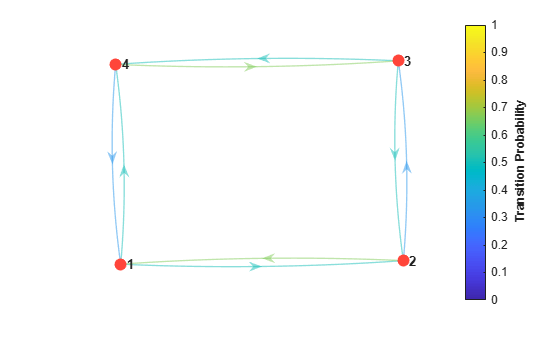Compute the expected first hitting time for state 1, beginning from each state in the Markov chain.

`ht = hittime(mc,1)`
```ht = 4×1 0 2.3333 4.0000 3.6667 ```

Plot a digraph of the Markov chain. Specify node colors representing the expected first hitting times for state 1, beginning from each state in the Markov chain.

`hittime(mc,1,'Graph',true);`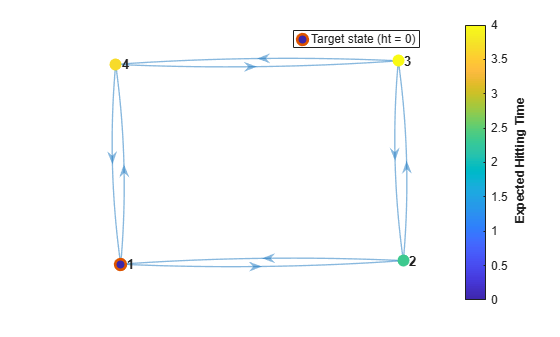Plot another digraph. Include state 4 as a target state.

`hittime(mc,[1 4],'Graph',true);`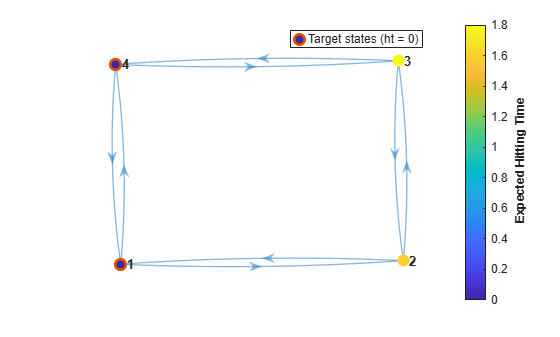Create the Markov chain characterized by this transition matrix:

`$P=\left[\begin{array}{ccccccc}1/2& 0& 1/2& 0& 0& 0& 0\\ 0& 1/3& 0& 2/3& 0& 0& 0\\ 1/4& 0& 3/4& 0& 0& 0& 0\\ 0& 2/3& 0& 1/3& 0& 0& 0\\ 1/4& 1/8& 1/8& 1/8& 1/4& 1/8& 0\\ 1/6& 1/6& 1/6& 1/6& 1/6& 1/6& 0\\ 1/2& 0& 0& 0& 0& 0& 1/2\end{array}\right].$`

```P = [1/2 0 1/2 0 0 0 0 0 1/3 0 2/3 0 0 0 1/4 0 3/4 0 0 0 0 0 2/3 0 1/3 0 0 0 1/4 1/8 1/8 1/8 1/4 1/8 0 1/6 1/6 1/6 1/6 1/6 1/6 0 1/2 0 0 0 0 0 1/2]; mc = dtmc(P);```

Compute the expected first hitting times for state 1, beginning from each state in the Markov chain `mc`. Also, plot a digraph and specify node colors representing the expected first hitting times for state 1.

`ht = hittime(mc,1,'Graph',true)`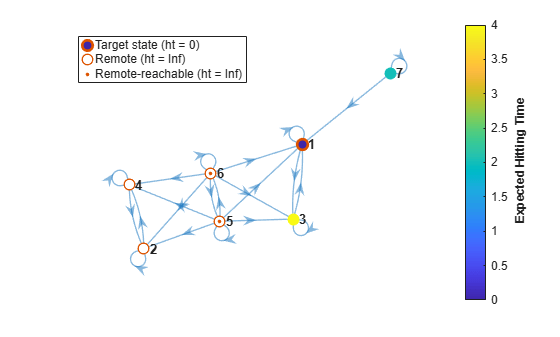```ht = 7×1 0 Inf 4 Inf Inf Inf 2 ```

States 2 and 4 form an absorbing class. Therefore, state 1 is unreachable from these states. The absorbing class is remote with respect to state 1, with an expected first hitting time of `Inf`.

State 1 is reachable from states 5 and 6, but the probability of transitioning into the absorbing class from states 5 and 6 is nonzero. Therefore, states 5 and 6 are remote-reachable with respect to state 1, with an expected first hitting time of `Inf`.

The expected first hitting time for state 1 beginning from state 7 is 2 time steps. The expected first hitting time for state 1 beginning from state 3 is 4 time steps.

Create an eight-state Markov chain from a randomly generated transition matrix with 50 infeasible transitions in random locations. An infeasible transition is a transition whose probability of occurring is zero. Assign arbitrary names to the states.

```numStates = 8; Zeros = 50; stateNames = strcat(repmat("Regime ",1,8),string(1:8)); rng(1617676169) % For reproducibility mc = mcmix(8,'Zeros',Zeros,'StateNames',stateNames);```

Plot a digraph of the Markov chain `mc`. Specify node colors that identify transient and recurrent states and communicating classes.

```figure; graphplot(mc,'ColorNodes',true);```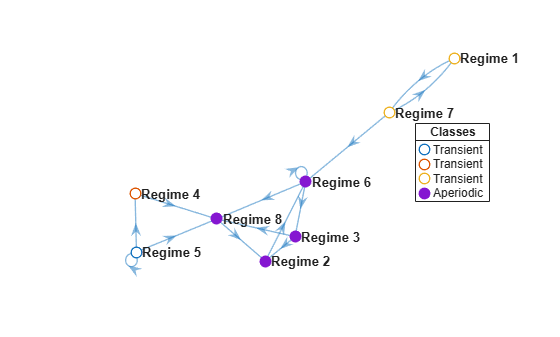Find a recurrent class in the Markov chain `mc` by following this procedure:

1. Classify the states by passing `mc` to `classify`. Return the array of class memberships `ClassStates` and the logical vector specifying whether the classes are recurrent `ClassRecurrence`.

2. Extract the recurrent classes from the array of classes by indexing into the array using the logical vector.

```[~,ClassStates,IsClassRecurrent] = classify(mc); recurrent = ClassStates{IsClassRecurrent}```
```recurrent = 1x4 string "Regime 2" "Regime 3" "Regime 6" "Regime 8" ```

Compute the expected hitting time for the states of the recurrent class, beginning from each state in the Markov chain.

`ht = hittime(mc,recurrent);`

Extract and display the expected absorption times beginning from the transient states.

```istransient = ~ismember(mc.StateNames,recurrent); absorbtime = ht(istransient); table(absorbtime,'RowNames',mc.StateNames(istransient))```
```ans=4×1 table absorbtime __________ Regime 1 5.8929 Regime 4 1 Regime 5 1.7777 Regime 7 4.8929 ```

Create a 50-state Markov chain from a random transition matrix in which most of the transitions are infeasible and randomly placed.

```rng(1) % For reproducibility mc = mcmix(50,'Zeros',2400);```

Visualize the mixing time of the Markov chain by plotting a digraph and specifying node colors representing the expected first hitting times for state 1, beginning from each state.

`hittime(mc,1,'Graph',true);`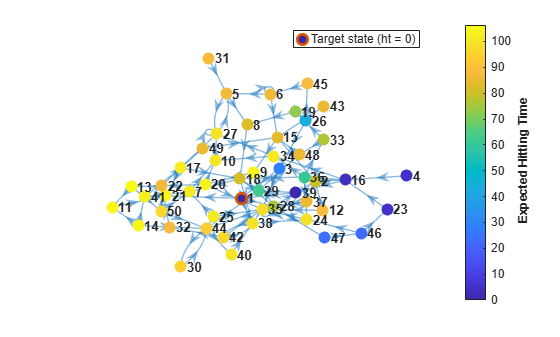Visualize the mixing time of the Markov chain for state 5.

`hittime(mc,5,'Graph',true);`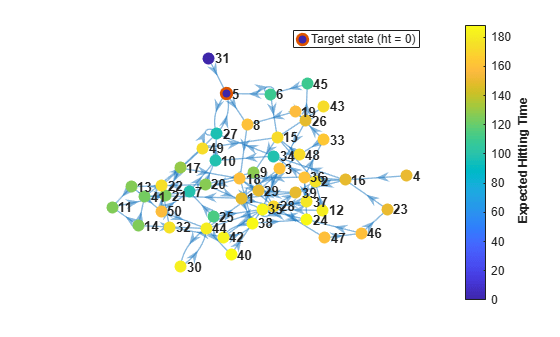The expected commute time from state $\mathit{i}$ to state $\mathit{j}$ is the expected time required for a Markov chain to transition from state $\mathit{i}$ to state $\mathit{j}$ (the expected first hitting time `ht`$\left(\mathit{i},\mathit{j}\right)$), then back to state $\mathit{i}$ (`ht`$\left(\mathit{j},\mathit{i}\right)$). Formally, the expected commute time is

$\mathit{C}\left(\mathit{i},\mathit{j}\right)=$`ht`$\left(\mathit{i},\mathit{j}\right)+\text{\hspace{0.17em}}$`ht`$\left(\mathit{j},\mathit{i}\right)$.

Create a "dumbbell" Markov chain containing 10 states in each "weight" and three states in the "bar."

• Specify random transition probabilities between states within each weight.

• If the Markov chain reaches the state in a weight that is closest to the bar, then specify a high probability of transitioning to the bar.

• Specify uniform transitions between states in the bar.

```rng(1); % For reproducibility w = 5; % Dumbbell weights DBar = [0 1 0; 1 0 1; 0 1 0]; % Dumbbell bar DB = blkdiag(rand(w),DBar,rand(w)); % Transition matrix % Connect dumbbell weights and bar DB(w,w+1) = 1; DB(w+1,w) = 1; DB(w+3,w+4) = 1; DB(w+4,w+3) = 1; mc = dtmc(DB);```

Plot a digraph of the Markov chain `mc`. Suppress node labels.

```figure; graphplot(mc);```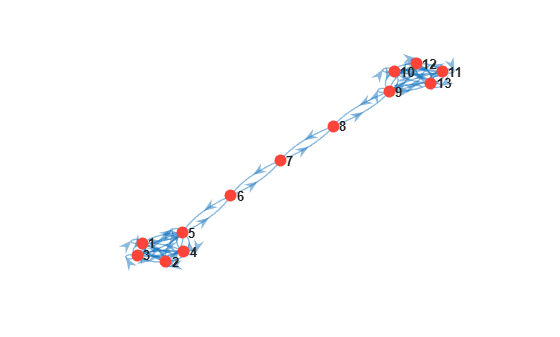Consider computing the expected commute time from state 1 to state 10.

Compute `ht`(1,10), the expected first hitting time for state 10 beginning from state 1.

```ht = hittime(mc,10); ht1to10 = ht(1);```

Compute `ht`(10,1), the expected first hitting time for state 1 beginning from state 10.

```ht = hittime(mc,1); ht10to1 = ht(10);```

Compute the expected commute time from state 1 to state 10.

`C1to10 = ht1to10 + ht10to1`
```C1to10 = 236.6165 ```

The expected commute time from state 1 to state 10 and back is 236.62 time steps.

## Input Arguments

collapse all

Discrete-time Markov chain with `NumStates` states and transition matrix `P`, specified as a `dtmc` object. `P` must be fully specified (no `NaN` entries).

Target subset of states, specified as a numeric vector of positive integers, string vector, or cell vector of character vectors.

• For a numeric vector, elements of `target` correspond to rows of the transition matrix `mc.P`.

• For a string vector or cell vector of character vectors, elements of `target` must be state names in `mc.StateNames`.

Example: `["Regime 1" "Regime 2"]`

Data Types: `double` | `string` | `cell`

Axes on which to plot, specified as an `Axes` object.

By default, `hittime` plots to the current axes (`gca`).

## Output Arguments

collapse all

Expected first hitting times, returned as a numeric vector of length `mc.NumStates`. `ht(j)` is the expected first hitting time of the specified subset of the target states `target` from starting state `j`.

If `ht(j)` = `Inf`, state `j` is a remote state or remote-reachable state for the target states `target`.

Handle to the graph plot, returned as a graphics object when the `'Graph'` name-value pair argument is `true`. `h` is a unique identifier, which you can use to query or modify properties of the plot.

collapse all

### Remote State

Remote states are those states from which the target states are unreachable. A remote state has a hitting probability of `0` and an expected first hitting time of `Inf`. For more details on hitting probabilities, see `hitprob`.

### Remote-Reachable State

Remote-reachable states are those states from which the target states are reachable and that have a positive probability of reaching an absorbing class. A remote-reachable state has an expected first hitting time of `Inf` for the target states.

## Algorithms

`hittime` uses `linprog` to find the minimum norm nonnegative solution to the system:

`$\left\{\begin{array}{cc}{k}_{i}^{A}=0& ,i\in A\\ {k}_{i}^{A}=1+\sum _{j\notin A}^{}{P}_{ij}{k}_{j}^{A}& ,i\notin A,\end{array}$`

where

• ${k}_{i}^{A}$ = `ht(i)`, the expected first hitting time for the subset of states A, beginning from state `i`.

• A is the set of indices of the states in `target`.

• P = `mc.P`.

• N = `mc.NumStates` .

 Norris, J. R. Markov Chains. Cambridge, UK: Cambridge University Press, 1997.